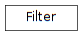# 4.3. pyopus.optimizer.filter — Filter-based point acceptance¶Filter support for constrained optimization (PyOPUS subsystem name: FILT)

class pyopus.optimizer.filter.Filter(hmax=0.0, debug=0)

All points are stored in a dictionary with keys of the form (f,h).

The value stored alongside (f,h) can be anything.

A point (f,h) dominates (f0,h0) if

• h<h0 and f<=f0 or
• h<=h0 and f<f0

(f,h) is dominated by the filter if h>hmax.

No point in the filter dominates any other point in the filter.

hmax=0 results in extreme barrier behavior.

accept(f, h, misc)

Checks a point against the filter.

If h>hmax the point does not dominate nor is dominated. Such a point is rejected.

If filter is empty, a point is accepted. Such a point is considered to dominate filter.

If point dominates any filter point it is accepted. Dominated filter points are deleted.

If a point is dominated by any filter point it is rejected. If a point does not dominate nor is dominated it is accepted.

Returns boolean tuple (dominates, dominated, accepted).

bestFeasible()

Returns (f,h,misc) of the feasible point.

Returns (None, None, None) if no such point exists.

static dominates(f, h, f0, h0)

Returns True if (f,h) dominates (f0,h0).

leastInfeasible()

Returns (f,h,misc) of the infeasible point with lowest h.

Returns (None, None, None) if no such point exists.

mostInfeasible()

Returns (f,h,misc) of the infeasible point with highest h.

Returns (None, None, None) if no such point exists.

orderedHlist()

Returns h values in increasing order.

orderedPoints()

Returns the f and h values ordered by increasing h.

position(f, h)

Returns the position of h in the ordered list of h values.

0 ... feasible point 1 ... best infeasible point 2 ... second infeasible point ...

Returns None if the point is not in the filter.

reset(hmax=None)

Resets the filter.

Points are stored in a dictionary with h as key. Dictionary values are tuples of the form (f, misc). There can be only one point for every h0 value.

updateHmax(hmax)

Updates hmax and purges points with h>hmax.

#### Previous topic

4.2. pyopus.optimizer.cache — Caching of function and constraint values, and annotations

#### Next topic

4.4. pyopus.optimizer.coordinate — Box constrained coordinate search optimizer